# Third Chapter Lesson-2: Conversion of Decimal number into Binary, Octal & Hexadecimal number.

## At the end of this lesson-

• 1. You will be able to convert a decimal number into binary number.
• 2. You will be able to convert a decimal number into octal number.
• 3. You will be able to convert a decimal number into hexadecimal number.

## Number System Conversions:

There are twelve sorts of conversions amoung four number systems. They are-## The same rules can be divided into the following ways-

Decimal Number System to Other Base

• Decimal Number System to Binary Number System
• Decimal Number System to Octal Number System
• Decimal Number System to Hexadecimal Number System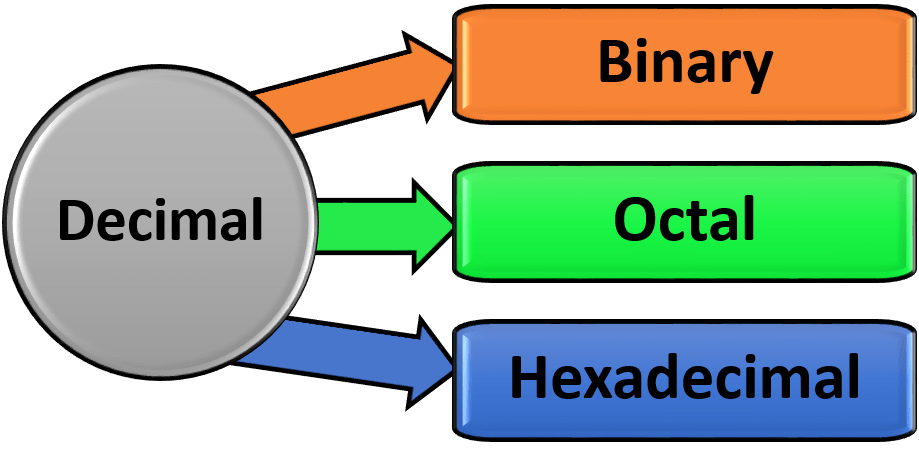Other Base to Decimal Number System

• Binary Number System to Decimal Number System
• Octal Number System to Decimal Number System
• Hexadecimal Number System to Decimal Number System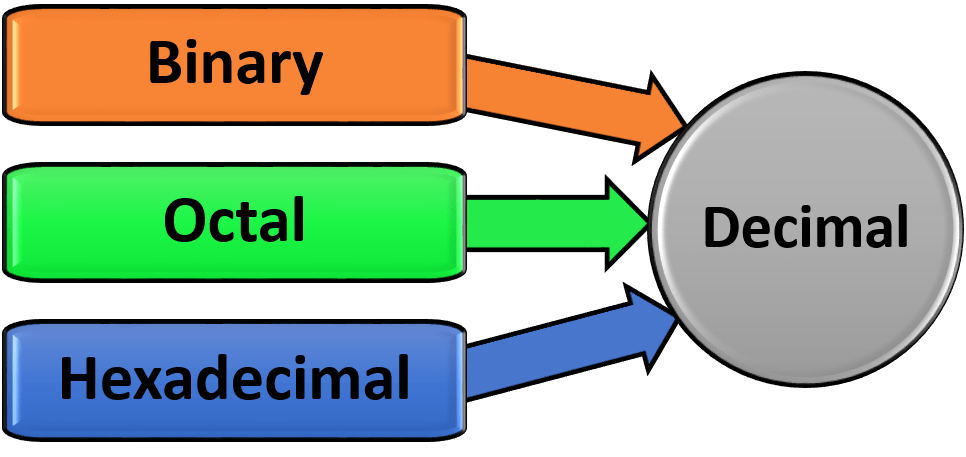Conversion among Binary, Octal & Hexadecimal or Non-decimal Number Systems

• Octal & Hexadecimal to Binary Number system
• Binary to Octal & Hexadecimal Number system
• Octal to Hexadecimal Number system
• Hexadecimal to Octal Number system
With the help of  conversion of the first and second groups, the conversion of this group can be done in two steps as in the diagram below-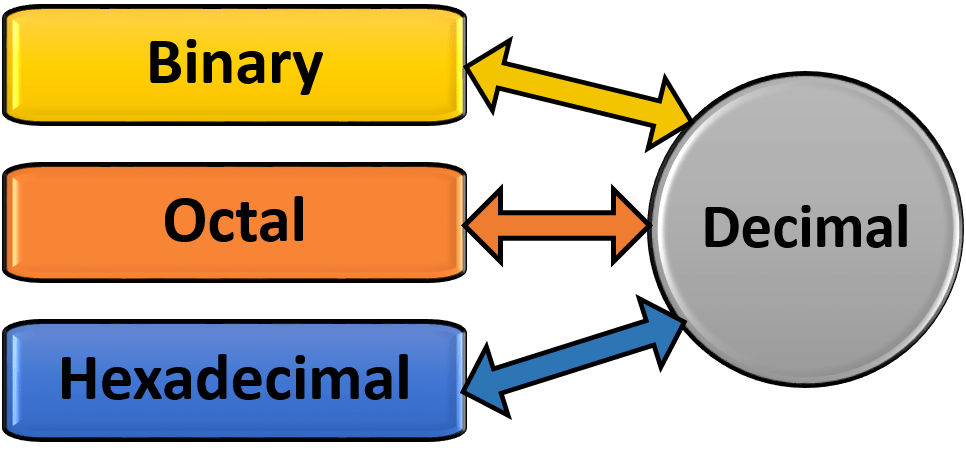In addition to the above methods It can be done in the following ways:## Decimal Number System to Other Base:### For Integer Part:

Step 1 − Divide the integer part of decimal number by the base of target number system (in which you want to convert the number: Binary (2), octal (8) and Hexadecimal (16)).

Step 2 − Get quotient and remainder from Step 1

Step 3 − Divide the quotient of Step 1 by the base of target number system again

Step 4 − Get quotient and remainder from Step 3

Repeat this procedure until the quotient becomes zero.

Traverse the remainders from bottom to top to obtain the target number system.

### For Fractional Part:

Step 1 − Multiply fractional part of the decimal number by the base of target number system (in which you want to convert the number: Binary (2), octal (8) and Hexadecimal (16)).

Step 2 −Write the integer part and fractional part of the product so obtained separately.

Step 3 − Multiply the fractional part of the previous product by the base of target number system.

Step 4 − Write the integer part and fractional part of the product so obtained separately.

Repeat this procedure until the fractional part remains 0.

[The fractional part does not terminates to 0 after several iterations.

So, let us find the value up to 4 decimal places.]

Traverse the real part column from top to bottom to obtain the target number system.

### Decimal Number System to Binary Number System:

Example: Convert (17)10 to Binary number system.So, (17)10 = (10001)2

Example: Convert (0.125)10 to Binary number system.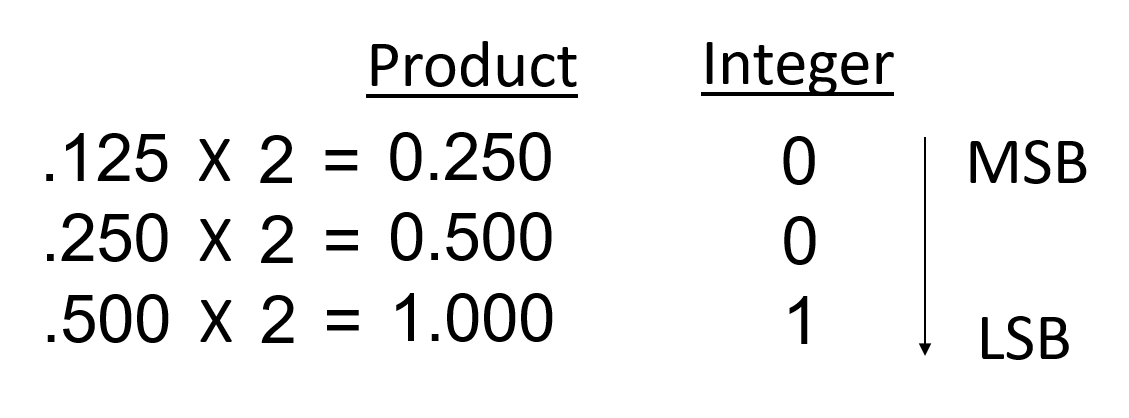So, (0.125)10 = (.001)2

• Convert (35.75)10 to Binary number system.
• Convert (75.69)10 to Binary number system.

### Decimal Number System to Octal Number System:

Example: Convert (423)10 to Octal number system.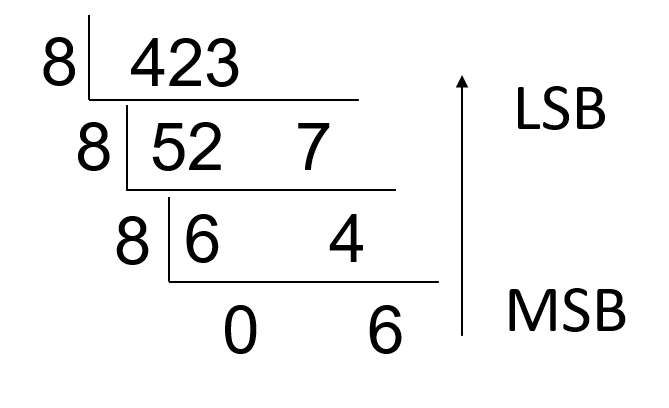So, (423)10 = (647)8

Example: Convert (.150)10 to Octal number system.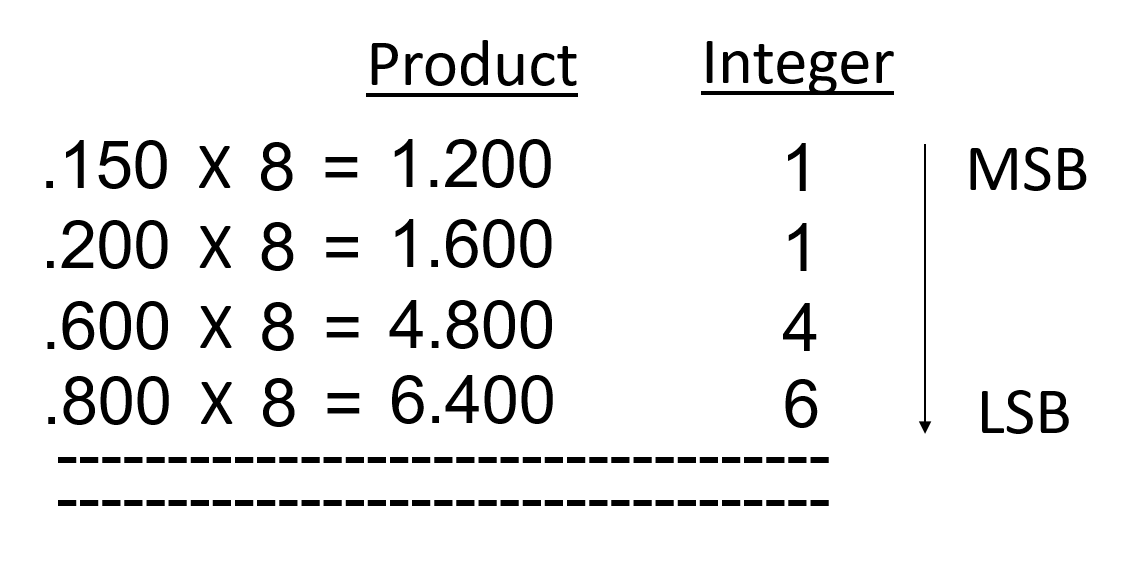So, (.150)10 = (.1146…..)8

• Convert (75.615)10 to Octal number system.
• Convert (755.150)10 to Octal number system.

### Decimal Number System to Hexadecimal Number System:

Example: Convert (423)10 to Hexadecimal number system.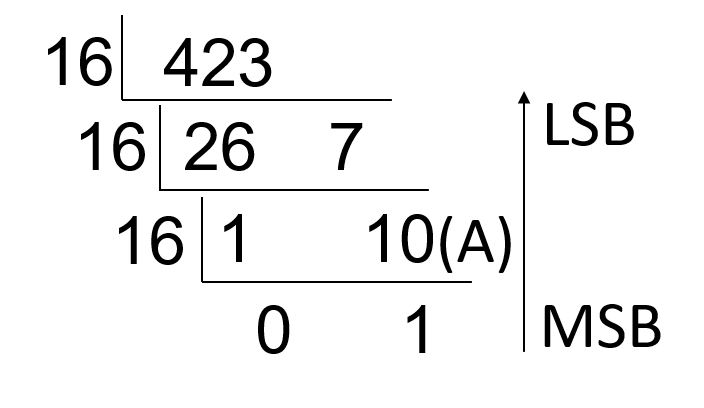So,  (423)10 = (1A7)16

Example: Convert (.150)10 to Hexadecimal number system.So, (.150)10 = (.266…..)16

• Convert (615.625)10 to Hexadecimal number system.
• Convert (125.150)10 to Hexadecimal number system.

## Lesson Evaluation-

Knowledge Based Questions:

Comprehension Based Questions:

Creative Questions:

ICT teacher was teaching number system in class XI. But he was irritated by a student’s inattentiveness and asked for his roll number. The student answered (31)10. Then the teacher asked the student’s last class roll and student answered (15)10. Then the teacher said to him, Your inattentiveness made your bad result.

c) Express the current class roll of the stimulus student in a binary number system.

Multiple Choice Questions:

1. Which one is equivalent binary to 99?

a) 1101011       b) 1010011     c)1100011        d)1101010

2. Which one is octal form of 91?

a) 133     b) 131     c) 331     d) 313

3. Which one is binary value of (37.125)?

a) 100101.01     b)100101.001      c)101001.01       d) 101001.001

4. Which one is equivalent hexadecimal value for (43962)10 ?

a) ABBA     b) DADA     c) ABC    d) DBC

Written by,